# Catch up on the latest AI articles# Superior To ViT! A New Underlying Model For Large-Scale CNNs! : InternImage

3 main points
✔️ CNN model with Deformable Convolution at its core achieves accuracy equal to or better than ViT in classification, detection, and segmentation!
✔️ Achieved an impressive 1st place in object detection and segmentation!
✔️ DCNv3, an improvement of DCNv2, expands the receptive field with fewer parameters of 3x3 kernel!

written by Wenhai WangJifeng DaiZhe ChenZhenhang HuangZhiqi LiXizhou ZhuXiaowei HuTong LuLewei LuHongsheng LiXiaogang WangYu Qiao
(Submitted on 10 Nov 2022 (v1), last revised 13 Nov 2022 (this version, v2))

Subjects: Computer Vision and Pattern Recognition (cs.CV)code：The images used in this article are from the paper, the introductory slides, or were created based on them.

## Introduction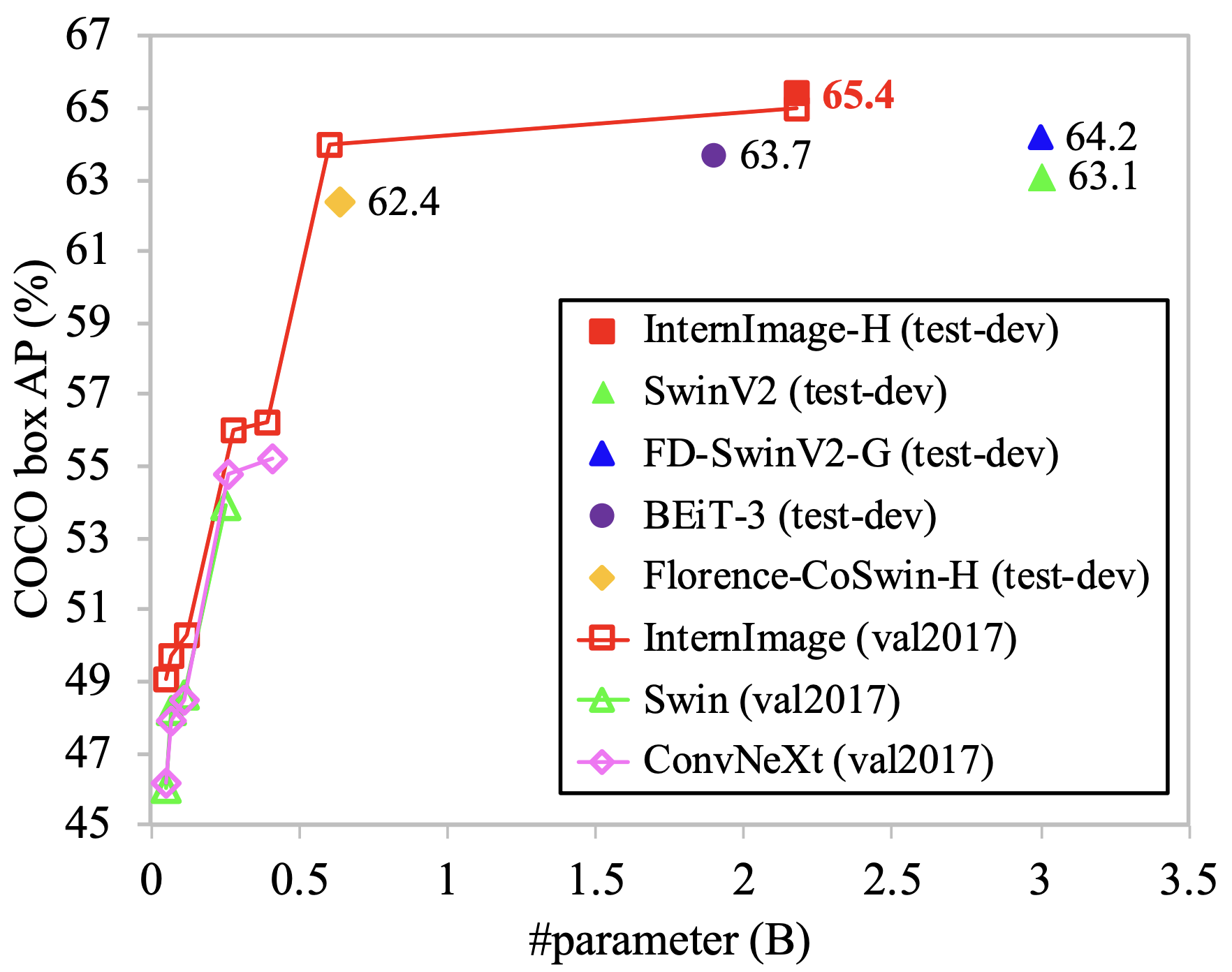The above figure shows the accuracy of ViT-based and CNN-based methods for object detection on the COCO dataset, as well as InternImage, which achieves the highest accuracy with the fewest parameters.

CNNs have dominated the field of ComputerVision since AlexNet, but today the Vision Transformer (ViT), which can learn large datasets without inductive bias, has taken over many tasks. The gap in superiority between CNN and ViT is twofold

• ViT can learn long-range dependencies without inductive bias
• ViT can acquire adaptive dependencies on inputs through dynamic weights

InternImage fills this gap by employing DCNs, enabling training on large datasets with fewer parameters and higher accuracy. Like ViT, we are testing models at a variety of scales. Let's take a look at InternImage.

## systematic positioning

The main difference between CNN and ViT is the presence of inductive bias: CNN assumes feature locality as a bias and employs a structure of multiple layers of local feature extraction with small kernels. Because of this structure, CNNs can be trained on relatively small data sets, but in general, the key to the high accuracy of deep learning models is to train on large data sets. which should be available from large datasets. ViT, on the other hand, is a long-range dependent mechanism that learns relationships between all local patches in the image without assuming inductive bias. While overlearning on small data is an issue, ViT has an overwhelming advantage when learning on large data.

Another difference is whether the weights are dynamic or static: CNNs use a unique learned kernel for feature extraction, independent of local patches, during inference. On the other hand, ViT dynamically acquires weights according to the dependencies to be extracted by linearly projecting local patches to Q, K, and V just before taking Attention and extracting relationships between patches with different weights depending on the input during inference.

These two are key to bridging the gap between ViT and CNNs. The most recently proposed large-scale CNN model, RepLKNet, focuses on the relationship between long-range dependence and effective receptive field and can train large data sets by incorporating large kernels. By incorporating feature re-parameterization and depth-wise convolution, we were able to successfully train kernels as large as 31x31. However, the paper points out that there is still a gap with ViT, and that there are many issues to be solved, such as the large 31x31 parameters and the complexity of the gimmick to make it trainable.

The proposed method, InternImage, solves the above two problems with a small 3x3 kernel and is proposed as the basis for a new CNN that can be efficiently scaled up. As with recent research on CNN scaling, it is constructed concerning various mechanisms of ViT.

## DCNv3

This paper focuses on Deformable Convolution Networks (DCN) to achieve long-range dependence and dynamic weights. The advantages are as follows

• Unlike conventional convolution, it can adaptively respond to long-range dependencies and inputs.
• Unlike MHSA, it inherits the inductive bias, so it can learn efficiently with less training data and time.
• Unlike MHSA and RepLKNet, the 3x3 kernel is complete and computationally and memory efficient

DCN is a convolution of a kernel that normally takes a rectangular window and can be transformed according to the data. See the figure below. The image below shows (a) local Attention, (b) global Attention, (c) a large kernel such as RepLKNet, and (d) DCN computation. is computationally expensive, and local attention does not capture long-range dependencies. While a large kernel can reduce the computational cost and capture long-range relationships compared to Attention, it cannot dynamically process the input. However, the DCN in (d) can capture the long-range relationship by deforming the 3x3 kernel with a small window, and the deformation and weights change adaptively depending on the input, thus achieving all the conditions of long-range dependence, dynamic weights, and computational cost.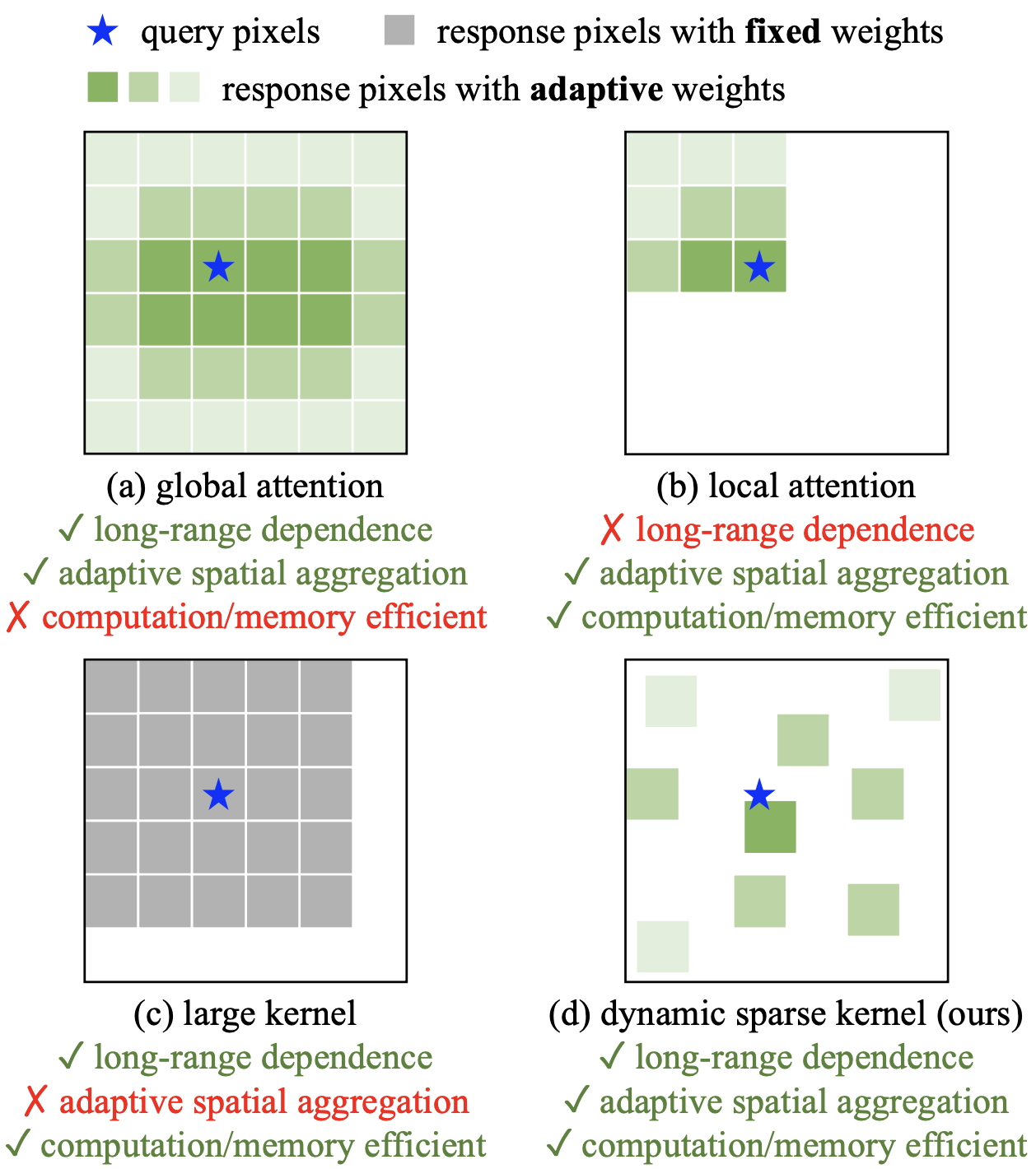### DCNv2

This paper proposes DCNv3, an improvement on the existing DCNv2, and adopts it for InternImage. DCNv2 is represented by the following equationIt shows the convolution of the input imagex, which is CxHxW, at coordinate p0. K is the number of parameters in the kernel, k is each square in the kernel, and pk is the coordinates in the image. The kernel weight wk is a static weight that has a common value regardless of the input coordinates, but DCN has Δp, which changes the coordinates to be weighted, and m, which weights x according to the input. This allows the receptive field and weights to change adaptively according to the shape and scale of the object in the picture. The argument should be indicated in the above equation because it depends on each coordinate p0. By involving x, Δp for 2xHxWandmfor 1xHxW is learned and output.DCNv3 makes the following changes to this

• Separation of depth-wise and point-wise convolution to reduce the computational cost
• Transformation and weighting from different perspectives by group convolution
• Normalize each m (p0)k in kernel units to stabilize learning

### Separable Convolution

Here is an illustration of Separable Convolution, where l is a layer. Convolution usually convolves the input spatial direction (each coordinate) and channel direction at a time and then calculates the convolution for Cl channels. On the other hand, Separable Convolution reduces the number of parameters significantly by separating the spatial convolution and channel convolution while maintaining the size of the output map. This is achieved by performing depth-wise convolution, which convolves only in the spatial direction, followed by point-wise convolution, which weights only between channels.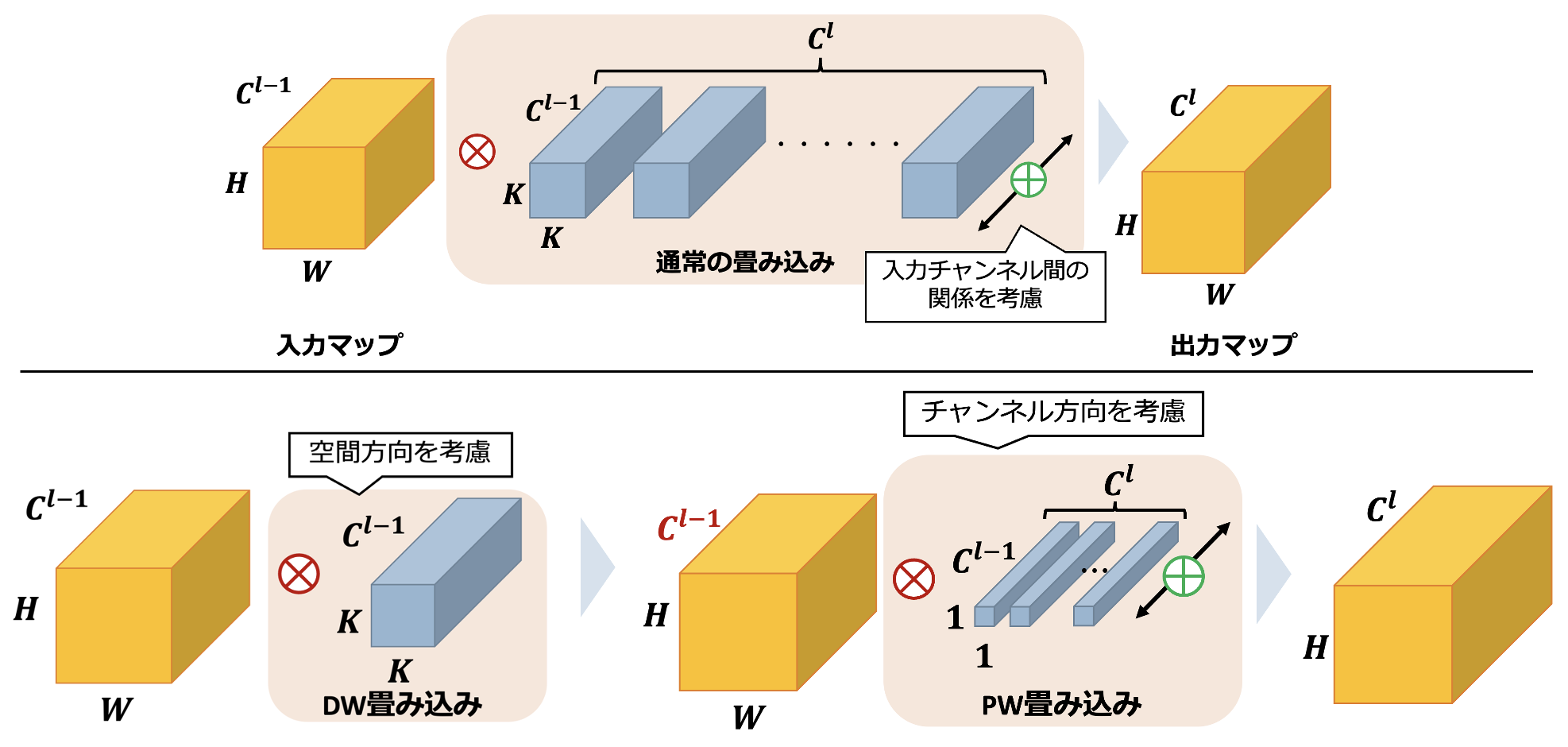### multigroup convolution

The kernel weight wk has a different viewpoint for each channel, but mk andΔpk is determined by the position of the input image and has a common value for all channels. DCNv3 is represented by the following equation, where each group of G is represented by g. The number of channels is not increased by G, but the input channel for each group is divided as C'=C/G. The xg in the equation represents it. The xg in the equation represents that.### Normalizedynamic weights m(p0)kin kernel units

In DCNv2, the sigmoid is applied so that m has a value of [0,1] for each coordinate. However, it has been pointed out that the sum of the weights in a kernel can vary greatly from 0 to K, making the learning process unstable. Therefore, DCNv3 employs a softmax function so that the sum of the weights is 1 for each kernel. This is expected to stabilize the learning process.

## InternImage

Now that we have grasped the advantages of DCNv3, we will build InternImage.Unlike ordinary CNNs, InternImage uses the ViT structure as a whole as a reference and adopts structures such as Layer Normalization, FFN, and GELU. This mechanism can be used for various image-processing tasks. This mechanism is effective in a variety of image-processing tasks.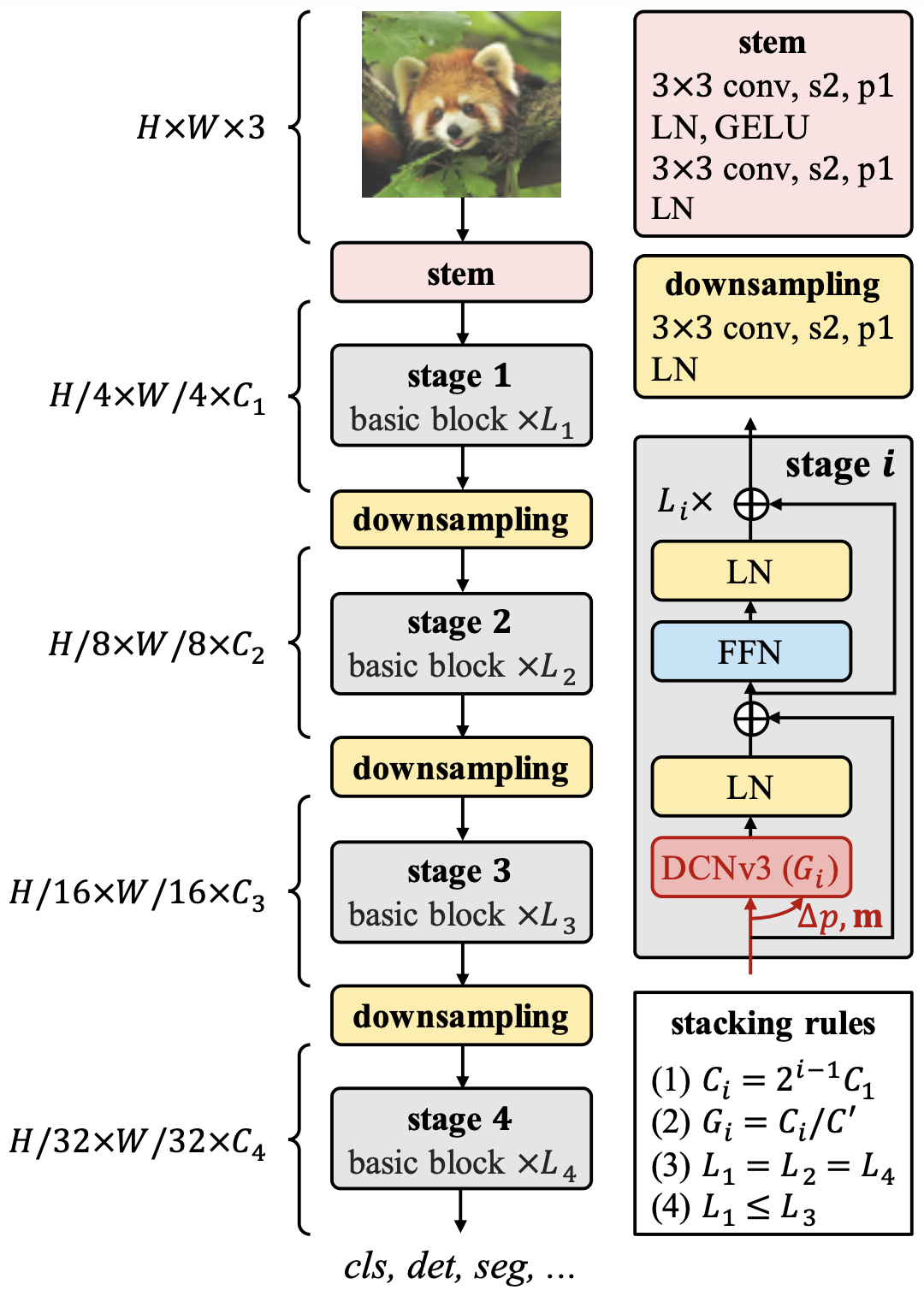### Stem, Downsampling, Stage

The stem is applied before the stage where DCNv3 is located; Stem consists of 3x3 convolution, LN, and GELU; RepLKNet has a similar structure, which explains the need to exhaustively extract local features in the early stages. At this stage, the resolution is reduced by a factor of 4. downsampling, as the name implies, is a block for downsampling by a factor of 2. The Stage contains DCNv3, the core of InternImage. The figure shows the most elementary base block, which is stacked to form each Stage.

### architecture

After Stem, the Stage and DownSampling are stacked four times. At each stage i, there are Ci channels, Li blocks, and Gi convolution groups. 12 hyperparameters are too large a search area, so three rules are established here to determine the structure and scaling.

1. The number of channels doubles based on the first stage C1.
2. The number of groups is determined by the number of channels Ci in each stage and the number of channels C' in each group
3. AABA" pattern with the same number of blocks per stage for stages 1, 2, and 4, and a large value for stage 3

In this way, the hyperparameters of the model are determined by (C1, C', L1, L3).

### scaling (e.g. in computer graphics)

Scale the model with (C1, C', L1, L3)=(64,16,4,18) totaling 30 million training parameters as InternImage-T. Increase the depth D(3xL1+L3) and the number of channels C1 by α=1.09,β=1.36,ΦThe resulting InternImage's various scales are shown in the table below. The -T to -XL has a computational complexity comparable to ConvNext, while InternImage-H, the largest scale, has 1 billion parameters.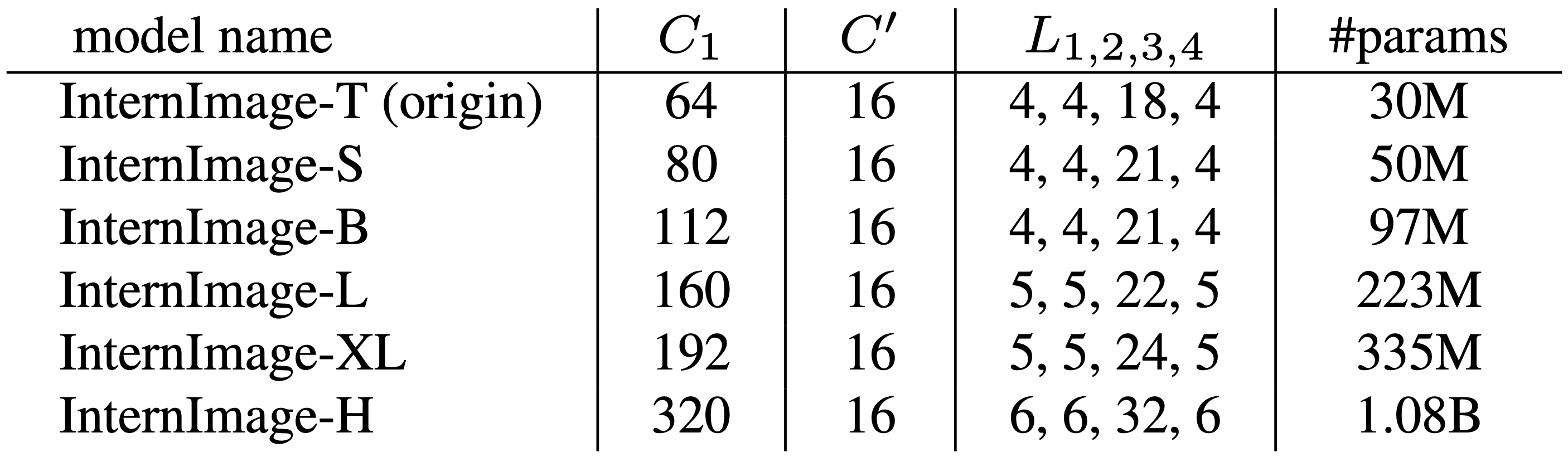## experiment

Experiments demonstrate the superiority of ImageNet's image classification, COCO's object detection using it as pre-training, and ADE20K's segmentation experiments. Each experiment compares CNN-based and VIT-based SoTA models at various scales. The ablation is included in the supplemental material, so those who want to know the superiority of detailed mechanisms should take a look at that as well.

To ensure fair comparisons that match the scale of the models being compared, -T/S/B is fine-tuning 1.3 million images of ImageNet-1K for 300 epochs, and -L/XL is fine-tuning 14.2 million images of ImageNet-22K for 30 epochs, which were pre-trained for 90 epochs. The large-scale -H is trained by M3I. For large-scale -H, M3I pre-training is employed. This method integrates supervised, weakly supervised, and unsupervised pre-training to obtain ideal parameters in only one stage of pre-training and a total of 427 million images (Laion-400M, YFCC-15M, and CC12M) are trained for 30 epochs. On top of that, 30 epochs of fine-tuning are performed on ImageNet-22K and -1K, respectively.

The following table shows the results, where the type is ViT(T) or CNN(C) and the scale is the input size. For each model scale, the results are comparable to or better than the CNN-based ViT SoTA models, with InternImage-T achieving the highest accuracy at 83.5%, and InternImage-S/B outperforming even the hybrid ViT CoAtNet-1. InternImage-XL and InternImage-H achieved 88.0% and 89.2%, respectively, better than conventional CNN models trained on the same large data set, and narrowing the gap with the large-scale ViT SoTA model to about 1.0. This result demonstrates the effectiveness of scaling and training on large data sets as defined earlier.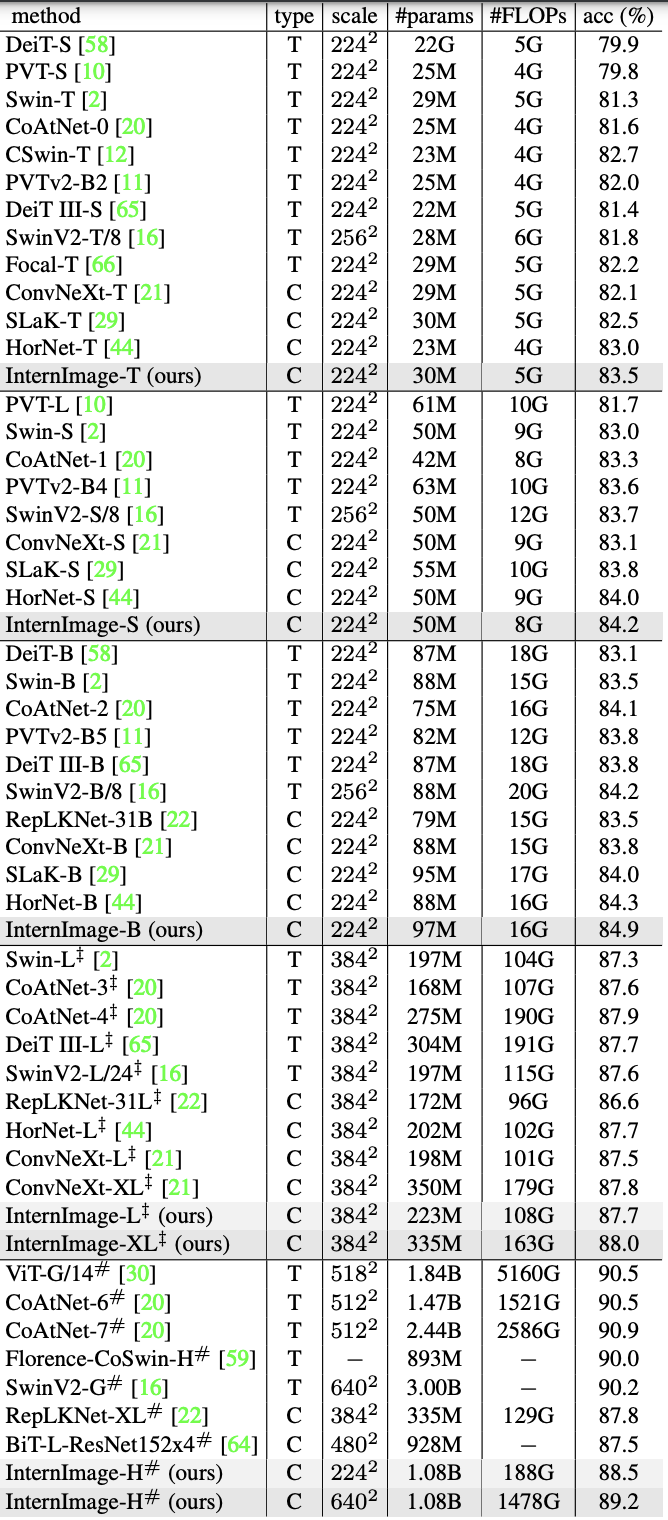### Object detection (COCO)

COCO has conducted two experiments to verify the accuracy: one comparing backbones using Mask R-CNN and Cascade Mask R-CNN as detectors, and the other comparing backbones using various detectors for each backbone. Each is fine-tuning a pre-trained version of ImageNet.

The following table compares the accuracy of training 12 epochs (1x) and 32 epochs (3x) in Mask R-CNN and Cascade Mask R-CNN, respectively. 3x training is performed multi-scale.

box-AP(APb): InternImage significantly outperforms Mask R-CNN with a similar number of parameters; at 1x, InternImage-T achieves an APb 4.5 higher than Swin-T and 3.0 higher than ConvNeXt-T. Cascade trained at 3x Mask R-CNN, InternImage-XL achieves an APb of 56.2, 1.0 higher than ConvNeXt-XL.

mask-AP(APm): InternImage-T achieved 42.5 in 1x training, outperforming SwinT and ConvNeXt. InternImage-XL's Cascade Mask R-CNN achieved the highest accuracy with 48.8 APm.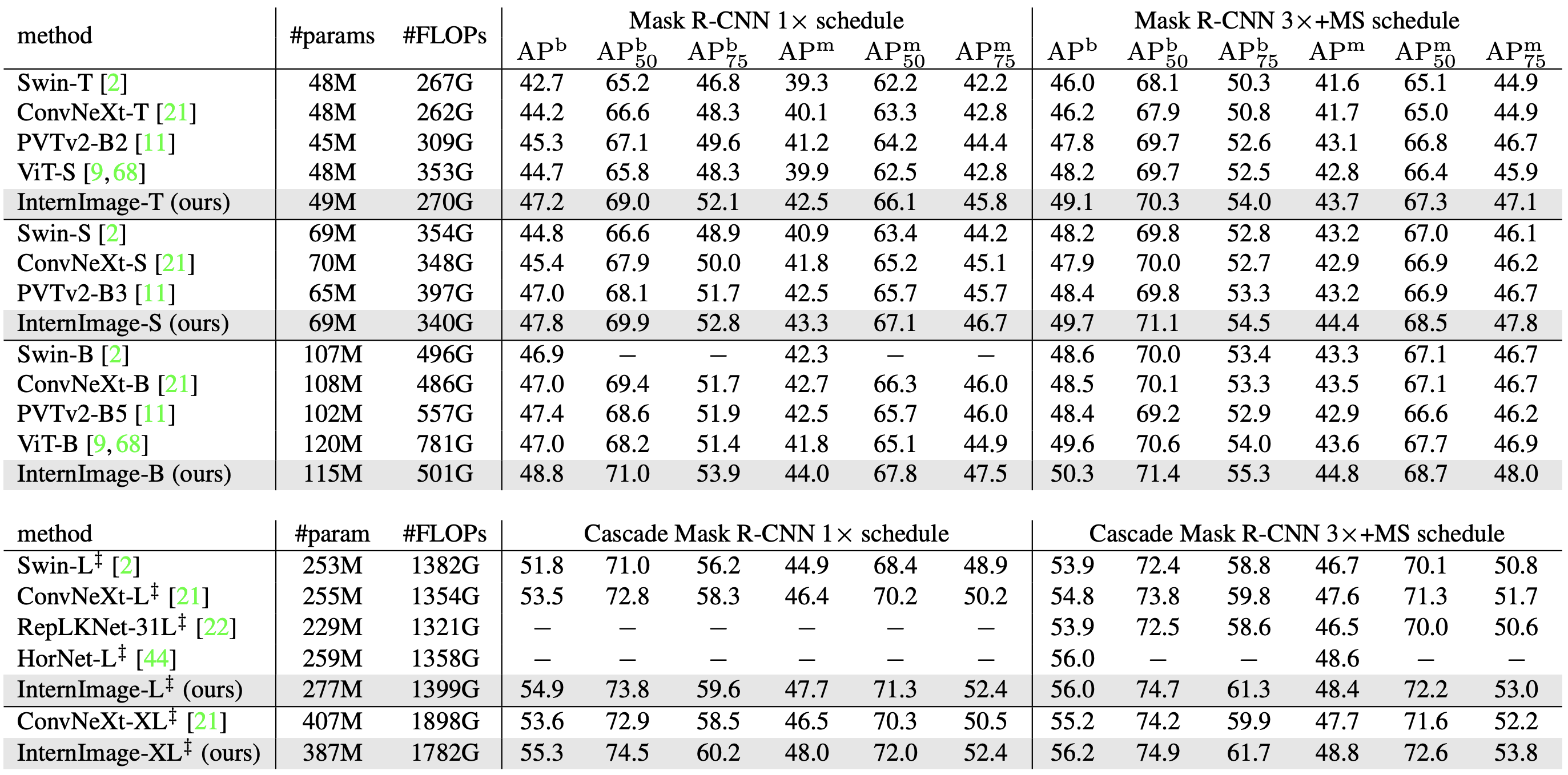SoTA Model

To further improve the accuracy, we employ a variety of detectors and compare their accuracy with the SoTA model. In this validation, the Objects365 dataset was trained for 26 epochs and COCO for 12 epochs. As a result, the DINO detector-based InternImage-XL/H achieved the highest accuracy of 65.0 APb and 65.4 APb on the val2017 and test-dev COCO datasets. Moreover, this was achieved with 27% fewer parameters than the existing SoTA model, demonstrating the effectiveness of InternImage for detection tasks.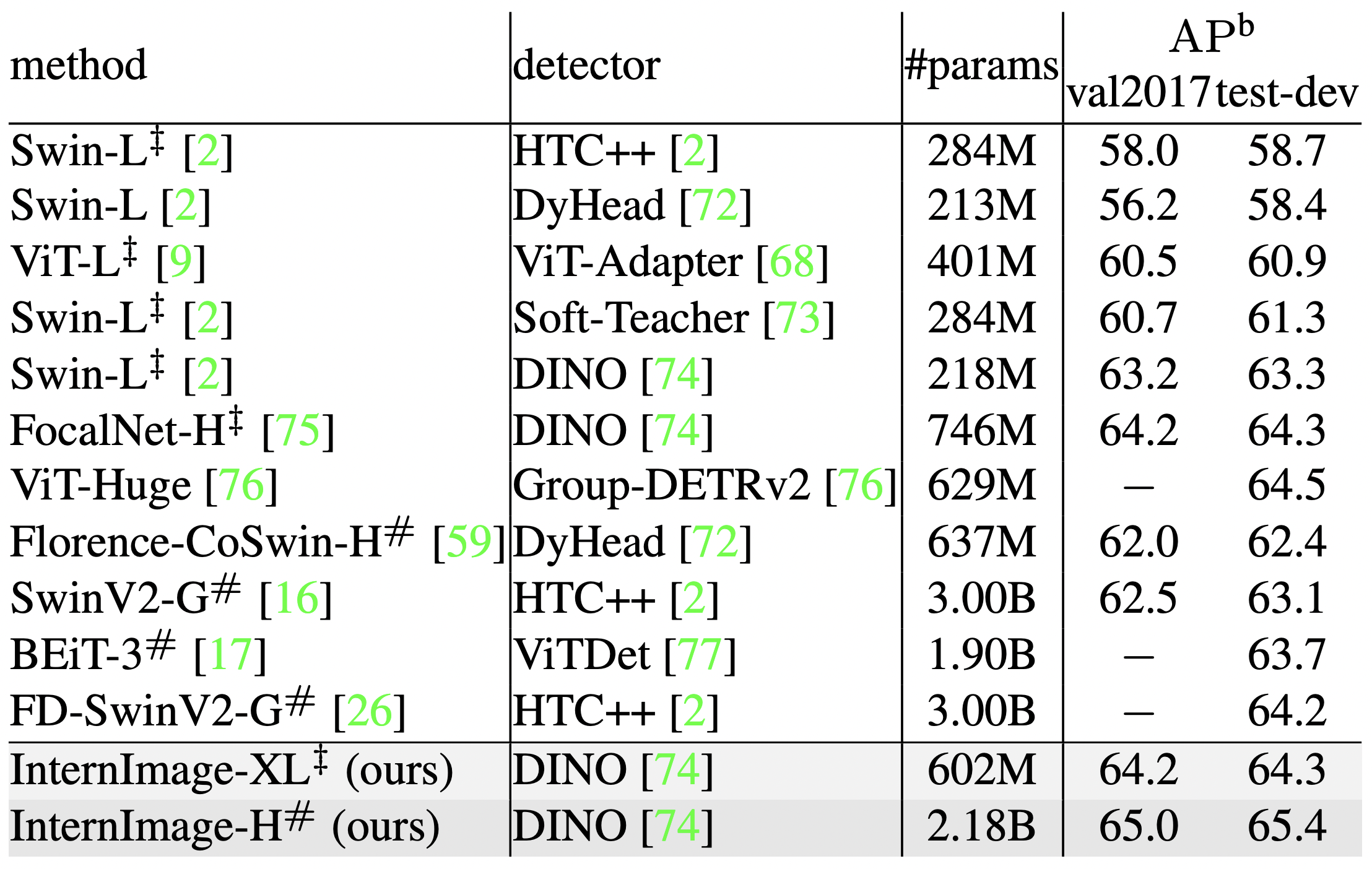### Object Detection (COCO) Semantic Segmentation (ADE20K)

As with detection, on top of ImageNet pre-training, UperNet is used as the model for the ADE20K segmentation task; InternImage-H is employed as the backbone for the more advanced model Mask2Former.

As a result, InternImage+UperNet consistently outperformed existing methods. With nearly the same number of parameters and FLOPs, InternImage-B achieved 50.8 mIoU, outperforming powerful CNN models such as ConvNeXt-B and RepLKNet-31B. At multiscale, InternImage-H achieved 62.9 mIoU, the highest accuracy in writing to date, outperforming the second-place BEiT-3.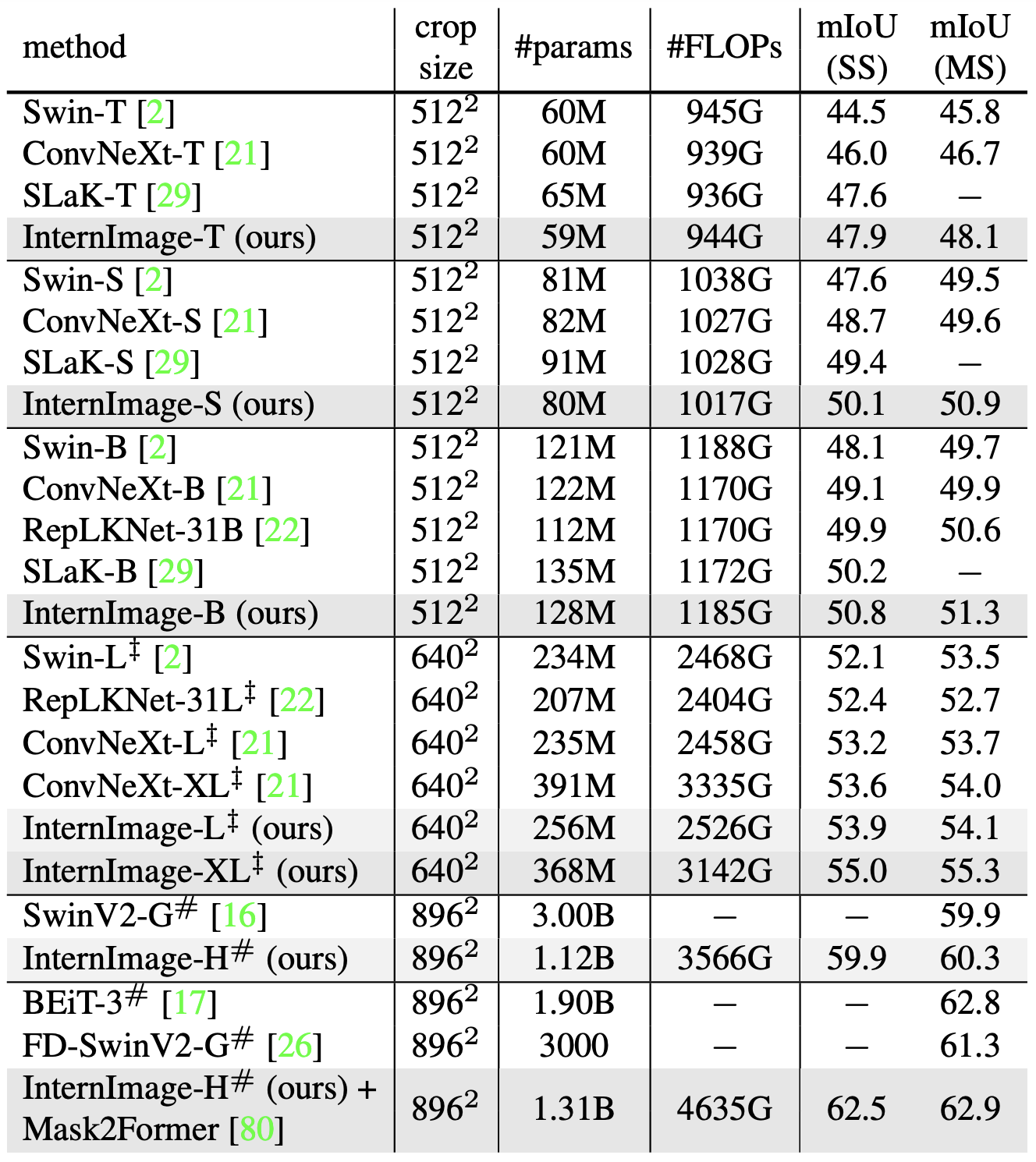These results show that the CNN-based base model can benefit from large data sets and comes very close to the ViT-based model.

## summary

In this presentation, we introduced InternImage, a large-scale CNN model that outperforms ViT. Experiments on ImageNet, COCO, ADE20K, and a wide range of benchmarks demonstrated that InternImage achieves accuracy comparable to or better than ViT trained on large data sets, showing that CNNs are a viable option for large-scale models. This demonstrates that CNNs have great potential as an option for large-scale models. One challenge is that DCN-based methods are processing-heavy. We look forward to future developments.

If you have any suggestions for improvement of the content of the article,